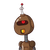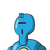# Sum of the areas of two squares is 468 m square if the difference of their perimeter is 24 m find the sides of the two square .​

Sum of the areas of two squares is 468 m square if the difference of their perimeter is 24 m find the sides of the two square .​

### 2 thoughts on “Sum of the areas of two squares is 468 m square if the difference of their perimeter is 24 m find the sides of the two square .​”

1.## ✎Δnsɯer࿐

Let the sides of the first and second square be X and Y .

Area of the first square = (X)²

Area of the second square = (Y)²

According to question,

(X)² + (Y)² = 468 m² ——(1).

Perimeter of first square = 4 × X and Perimeter of second square = 4 × Y

According to question,

4X – 4Y = 24 ——–(2)

From equation (2) we get,

4X – 4Y = 244X–4Y=24

4(X-Y) = 244(X−Y)=24

X – Y = 24/4X–Y=24/4

X – Y = 6X–Y=6

X=6+Y———(3)

Putting the value of X in equation (1)

(X)² + (Y)² = 468(X)²+(Y)²=468

= >  (6+Y)² + (Y)² = 468

= > (6)² + (Y)² + 2 × 6 × Y + (Y)² = 468

= > 36 + Y² + 12Y + Y² = 468

= > 2( Y² + 6Y – 216) = 0

= > Y² + 6Y – 216 = 0

= > Y² + 18Y – 12Y -216 = 0

= > Y(Y+18) – 12(Y+18) = 0

= > (Y+18) (Y-12) = 0

Putting Y = 12 in EQUATION (3)

X = 6+Y = 6+12 = 18X=6+Y=6+12=18

Side of first square = X = 18 m

Side of second square = Y = 12 m.

2.Let the sides of the first and second square be X and Y . Area of the first square = (X)²

Area of the second square = (Y)²

According to question, (X)² + (Y)² = 468 m² ——(1).

Perimeter of first square = 4 × X and Perimeter of second square = 4 × Y

According to question,

4X – 4Y = 24 ——–(2)

From equation (2) we get,

4X – 4Y = 24, 4(X-Y) = 24

X – Y = 24/4 , X – Y = 6

X = 6+Y ———(3)

Putting the value of X in equation (1)

(X)² + (Y)² = 468, (6+Y)² + (Y)² = 468

(6)² + (Y)² + 2 × 6 × Y + (Y)² = 468

36 + Y² + 12Y + Y² = 468

2Y² + 12Y – 468 +36 = 0

2Y² + 12Y -432 = 0

2( Y² + 6Y – 216) = 0

Y² + 6Y – 216 = 0

Y² + 18Y – 12Y -216 = 0

Y(Y+18) – 12(Y+18) = 0 (Y+18) (Y-12) = 0

(Y+18) = 0 Or (Y-12) = 0 Y = -18 OR Y = 12

Putting Y = 12 in EQUATION (3)

X = 6+Y = 6+12 = 18

Side of first square = X = 18 m

Side of second square = Y = 12 m.

[tex]\huge{\tt{\red{S}\green{H}\purple{I}\pink{N}\blue{C}\orange{H}\red{A} \green{N} \: \pink{✿༉}}}[/tex]